Name:    CIrcle Quiz

True/False
Indicate whether the statement is true or false.

1.

The circle with a centre ofand passing through the point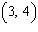has a radius of.

2.

The equation of the circle with a centre of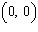and a radius measuring 6 units is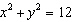.

Multiple Choice
Identify the choice that best completes the statement or answers the question.

3.

The point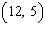is located on the circumference of the circle. What is the value of?
 a. 13 c. 144 b. 25 d. 169

4.

Which one of the following points lies on the circle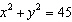?
 a.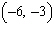c.b.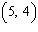d.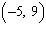5.

A circle passes through the points P, Q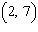, and R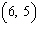. Which of the following points is at the centre of the circle?
 a.c.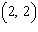b.d.6.

The circle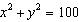has a chord joining the points P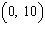and Q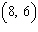. What is the equation of the right bisector of this chord?
 a.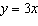c.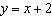b.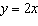d.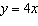7.

The points P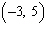and Q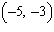lie on which of the circles?
 a.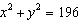c.b.d.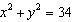8.

Which of the following points is the centre of the circle passing through the points A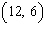, B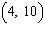, and C?
 a.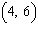c.b.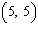d.